Please enable Javascript for your browser if it supports it or use the Browser that supports Javascript.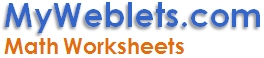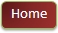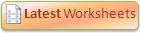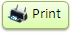Hide Answer Key Want to get notified about new worksheets?

 www.MyWeblets.com/kids/mathWorksheet - Mathematics
 Name:_____________________ Date: __________ Start Time: _________ Finish Time: _________

Calculate Mean, Median, Mode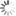Loading! Please Wait ...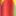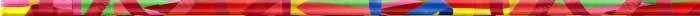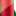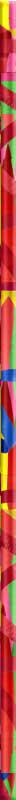[ Examples with steps ]:
 Calculate Mean for 1, 1, 2, 4, 7 (Sample A) Calculate Median for 1, 1, 2, 4, 7 (Sample C-1) Step 1: Compute the sum of all the terms. i.e. 1 + 1 + 2 + 4 + 7  = 15 Step 2: Count the terms. i.e. 5 Step 3: Compute the Mean or Average by dividing the sum with the count. i.e. 15 / 5 = 3 Step 1: Count the terms and find if the count is even or odd. Here Count is 5 and its odd. Step 2: Make sure that the terms are properly sequenced. Step 3: For odd count, Median is the middle term i.e. 2 Calculate Mode for 1, 1, 1, 2, 4, 7, 7 (Sample B) Calculate Median for 1, 1, 2, 4, 7, 8 (Sample C-2) Step 1: Find the terms that repeat more than one time. i.e 1 repeats three times and 7 repeats 2 times. Step 2: Mode is the term that repeat the most from these terms. i.e. since 1 repeats that most times, mode is 1 Note: If no term repeats, there is no mode. Step 1: Count the terms and find if the count is even or odd. Here Count is 6 and its even. Step 2: Make sure that the terms are properly sequenced. Step 3: For even count, Median is the sum of the middle pair divide by 2    (a) Find the middle pair i.e. 2, 4    (b) Add the numbers in the middle pair i.e. 2 + 4  = 6    (c) Median is i.e. 6 / 2 = 3

(a) 1, 2, 3, 3, 3, 4, 5

 Mean = Median = Mode =

(b) 1, 2, 3, 3, 4, 5

 Mean = Median = Mode =

(c) 1, 2, 2, 4, 4, 5

 Mean = Median = Mode =

(d) 1, 2, 3, 4, 5

 Mean = Median = Mode =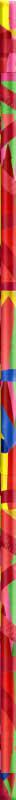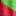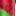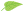Answer Key: (Cut the Answer Key or Fold It) Use "Hide Answer Key" option at the top if needed. (a) Mean:     3 Median:     3 Mode: 3 (b) Mean:     3 Median:   3  Mode: 3 (c) Mean:     3 Median:   3  Mode: 2, 4 (d) Mean:     3 Median:   3  Mode: None